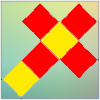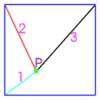# Search by Topic

#### Resources tagged with Pythagoras' theorem similar to 2D-3D:

Filter by: Content type:
Age range:
Challenge level:

### There are 79 results

Broad Topics > Pythagoras and Trigonometry > Pythagoras' theorem### Ball Packing

##### Age 14 to 16 Challenge Level:

If a ball is rolled into the corner of a room how far is its centre from the corner?### Spherical Triangles on Very Big Spheres

##### Age 16 to 18 Challenge Level:

Shows that Pythagoras for Spherical Triangles reduces to Pythagoras's Theorem in the plane when the triangles are small relative to the radius of the sphere.### In a Spin

##### Age 14 to 16 Challenge Level:

What is the volume of the solid formed by rotating this right angled triangle about the hypotenuse?### Circumnavigation

##### Age 14 to 16 Challenge Level:

The sides of a triangle are 25, 39 and 40 units of length. Find the diameter of the circumscribed circle.### Where Is the Dot?

##### Age 14 to 16 Challenge Level:

A dot starts at the point (1,0) and turns anticlockwise. Can you estimate the height of the dot after it has turned through 45 degrees? Can you calculate its height?### Reach for Polydron

##### Age 16 to 18 Challenge Level:

A tetrahedron has two identical equilateral triangles faces, of side length 1 unit. The other two faces are right angled isosceles triangles. Find the exact volume of the tetrahedron.### Star Gazing

##### Age 14 to 16 Challenge Level:

Find the ratio of the outer shaded area to the inner area for a six pointed star and an eight pointed star.### Retracircles

##### Age 16 to 18 Challenge Level:

Four circles all touch each other and a circumscribing circle. Find the ratios of the radii and prove that joining 3 centres gives a 3-4-5 triangle.### Get Cross

##### Age 14 to 16 Challenge Level:

A white cross is placed symmetrically in a red disc with the central square of side length sqrt 2 and the arms of the cross of length 1 unit. What is the area of the disc still showing?### Strange Rectangle

##### Age 16 to 18 Challenge Level:

ABCD is a rectangle and P, Q, R and S are moveable points on the edges dividing the edges in certain ratios. Strangely PQRS is always a cyclic quadrilateral and you can find the angles.### Ladder and Cube

##### Age 14 to 16 Challenge Level:

A 1 metre cube has one face on the ground and one face against a wall. A 4 metre ladder leans against the wall and just touches the cube. How high is the top of the ladder above the ground?### Belt

##### Age 16 to 18 Challenge Level:

A belt of thin wire, length L, binds together two cylindrical welding rods, whose radii are R and r, by passing all the way around them both. Find L in terms of R and r.### Baby Circle

##### Age 16 to 18 Challenge Level:

A small circle fits between two touching circles so that all three circles touch each other and have a common tangent? What is the exact radius of the smallest circle?### Compare Areas

##### Age 14 to 16 Challenge Level:

Which has the greatest area, a circle or a square inscribed in an isosceles, right angle triangle?### Two Circles

##### Age 14 to 16 Challenge Level:

Draw two circles, each of radius 1 unit, so that each circle goes through the centre of the other one. What is the area of the overlap?### Squ-areas

##### Age 14 to 16 Challenge Level:

Three squares are drawn on the sides of a triangle ABC. Their areas are respectively 18 000, 20 000 and 26 000 square centimetres. If the outer vertices of the squares are joined, three more. . . .### Pythagoras for a Tetrahedron

##### Age 16 to 18 Challenge Level:

In a right-angled tetrahedron prove that the sum of the squares of the areas of the 3 faces in mutually perpendicular planes equals the square of the area of the sloping face. A generalisation. . . .### The Dodecahedron

##### Age 16 to 18 Challenge Level:

What are the shortest distances between the centres of opposite faces of a regular solid dodecahedron on the surface and through the middle of the dodecahedron?### Circle Packing

##### Age 14 to 16 Challenge Level:

Equal circles can be arranged so that each circle touches four or six others. What percentage of the plane is covered by circles in each packing pattern? ...### Under the Ribbon

##### Age 14 to 16 Challenge Level:

A ribbon is nailed down with a small amount of slack. What is the largest cube that can pass under the ribbon ?### Are You Kidding

##### Age 14 to 16 Challenge Level:

If the altitude of an isosceles triangle is 8 units and the perimeter of the triangle is 32 units.... What is the area of the triangle?### About Pythagorean Golden Means

##### Age 16 to 18

What is the relationship between the arithmetic, geometric and harmonic means of two numbers, the sides of a right angled triangle and the Golden Ratio?### Squaring the Circle and Circling the Square

##### Age 14 to 16 Challenge Level:

If you continue the pattern, can you predict what each of the following areas will be? Try to explain your prediction.### Grid Lockout

##### Age 14 to 16 Challenge Level:

What remainders do you get when square numbers are divided by 4?### Holly

##### Age 14 to 16 Challenge Level:

The ten arcs forming the edges of the "holly leaf" are all arcs of circles of radius 1 cm. Find the length of the perimeter of the holly leaf and the area of its surface.### Circle Scaling

##### Age 14 to 16 Challenge Level:

Describe how to construct three circles which have areas in the ratio 1:2:3.### Inscribed in a Circle

##### Age 14 to 16 Challenge Level:

The area of a square inscribed in a circle with a unit radius is, satisfyingly, 2. What is the area of a regular hexagon inscribed in a circle with a unit radius?### Slippage

##### Age 14 to 16 Challenge Level:

A ladder 3m long rests against a wall with one end a short distance from its base. Between the wall and the base of a ladder is a garden storage box 1m tall and 1m high. What is the maximum distance. . . .### Classic Cube

##### Age 16 to 18 Challenge Level:

The net of a cube is to be cut from a sheet of card 100 cm square. What is the maximum volume cube that can be made from a single piece of card?### Where to Land

##### Age 14 to 16 Challenge Level:

Chris is enjoying a swim but needs to get back for lunch. If she can swim at 3 m/s and run at 7m/sec, how far along the bank should she land in order to get back as quickly as possible?### Incircles Explained

##### Age 16 to 18

This article is about triangles in which the lengths of the sides and the radii of the inscribed circles are all whole numbers.### The Fire-fighter's Car Keys

##### Age 14 to 16 Challenge Level:

A fire-fighter needs to fill a bucket of water from the river and take it to a fire. What is the best point on the river bank for the fire-fighter to fill the bucket ?.### Far Horizon

##### Age 14 to 16 Challenge Level:

An observer is on top of a lighthouse. How far from the foot of the lighthouse is the horizon that the observer can see?### Partly Circles

##### Age 14 to 16 Challenge Level:

What is the same and what is different about these circle questions? What connections can you make?### Six Discs

##### Age 14 to 16 Challenge Level:

Six circular discs are packed in different-shaped boxes so that the discs touch their neighbours and the sides of the box. Can you put the boxes in order according to the areas of their bases?### Semi-square

##### Age 14 to 16 Challenge Level:

What is the ratio of the area of a square inscribed in a semicircle to the area of the square inscribed in the entire circle?### Some(?) of the Parts

##### Age 14 to 16 Challenge Level:

A circle touches the lines OA, OB and AB where OA and OB are perpendicular. Show that the diameter of the circle is equal to the perimeter of the triangle### Equilateral Areas

##### Age 14 to 16 Challenge Level:

ABC and DEF are equilateral triangles of side 3 and 4 respectively. Construct an equilateral triangle whose area is the sum of the area of ABC and DEF.### The Medieval Octagon

##### Age 14 to 16 Challenge Level:

Medieval stonemasons used a method to construct octagons using ruler and compasses... Is the octagon regular? Proof please.### The Pillar of Chios

##### Age 14 to 16 Challenge Level:

Semicircles are drawn on the sides of a rectangle. Prove that the sum of the areas of the four crescents is equal to the area of the rectangle.### 30-60-90 Polypuzzle

##### Age 16 to 18 Challenge Level:

Re-arrange the pieces of the puzzle to form a rectangle and then to form an equilateral triangle. Calculate the angles and lengths.### Three Four Five

##### Age 14 to 16 Challenge Level:

Two semi-circles (each of radius 1/2) touch each other, and a semi-circle of radius 1 touches both of them. Find the radius of the circle which touches all three semi-circles.### Napkin

##### Age 14 to 16 Challenge Level:

A napkin is folded so that a corner coincides with the midpoint of an opposite edge . Investigate the three triangles formed .### Square World

##### Age 16 to 18 Challenge Level:

P is a point inside a square ABCD such that PA= 1, PB = 2 and PC = 3. How big is angle APB ?### Orthogonal Circle

##### Age 16 to 18 Challenge Level:

Given any three non intersecting circles in the plane find another circle or straight line which cuts all three circles orthogonally.### Medallions

##### Age 14 to 16 Challenge Level:

Three circular medallions fit in a rectangular box. Can you find the radius of the largest one?### Rectangular Pyramids

##### Age 14 to 18 Challenge Level:

Is the sum of the squares of two opposite sloping edges of a rectangular based pyramid equal to the sum of the squares of the other two sloping edges?### Take a Square

##### Age 14 to 16 Challenge Level:

Cut off three right angled isosceles triangles to produce a pentagon. With two lines, cut the pentagon into three parts which can be rearranged into another square.### Semi-detached

##### Age 14 to 16 Challenge Level:

A square of area 40 square cms is inscribed in a semicircle. Find the area of the square that could be inscribed in a circle of the same radius.### Incircles

##### Age 16 to 18 Challenge Level:

The incircles of 3, 4, 5 and of 5, 12, 13 right angled triangles have radii 1 and 2 units respectively. What about triangles with an inradius of 3, 4 or 5 or ...?